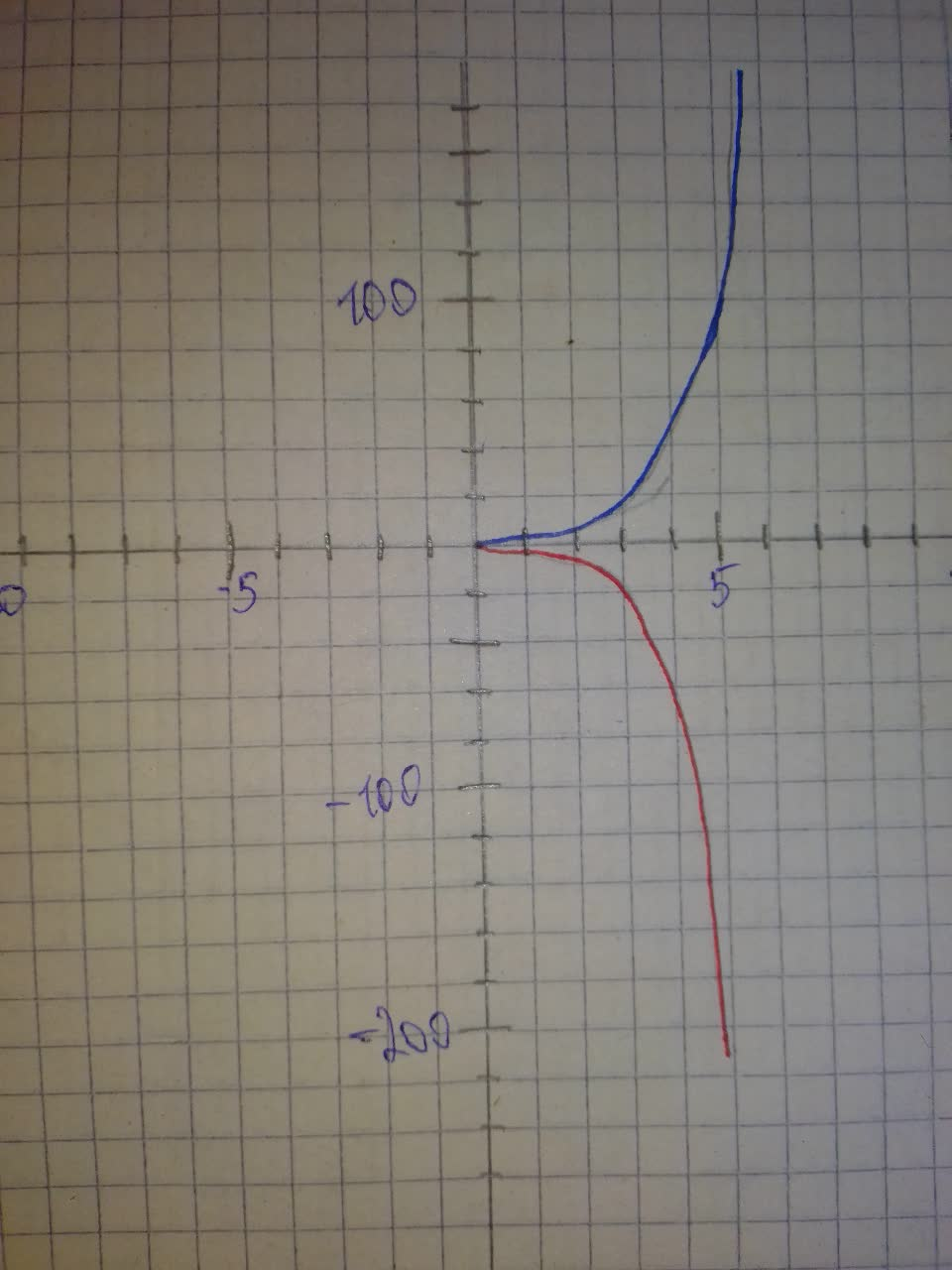# Graph f and g in the same rectangular coordinate system.Ernstfalld 2021-08-08 Answered
Graph f and g in the same rectangular coordinate system. Use transformations of the graph of f to obtain the graph of g. Graph and give equations of all asymptotes. Use the graphs to determine each functions
You can still ask an expert for help

• Questions are typically answered in as fast as 30 minutes

Solve your problem for the price of one coffee

• Math expert for every subject
• Pay only if we can solve itCullenThe graph for $-{3}^{x}$ is a reflection about the x-axis of ${3}^{x}$.
The domain is all real numbers.
The range for ${3}^{x}$ is all nonnegative numbers.
The range for ${3}^{-x}$ is all nonpositive numbers.
The horizontal asymptote for both functions is $y=0$.